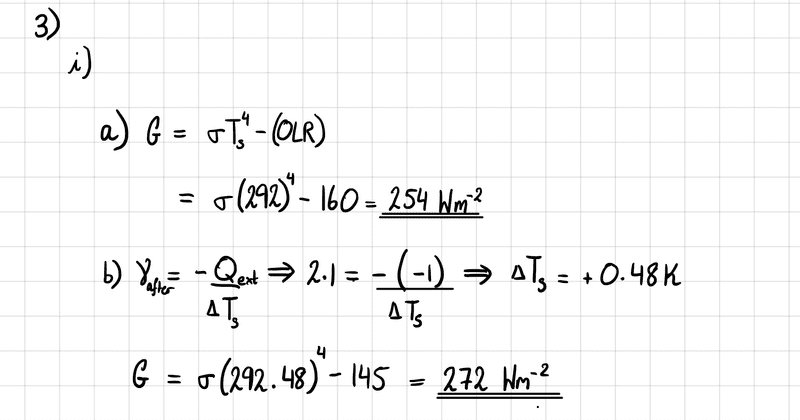# Environmental Physics - Radiative forcing effect on greenhouse effect

ProbablySid
Homework Statement:
To mitigate climate change, a large-scale afforestation project is commenced. The project aims to create a radiative forcing of ##\Delta Q = -1.0 Wm^{-2}## over 50 years by the removal of ##CO_{2}## through photosynthesis. Before the project is undertaken, a typical value of the outgoing longwave radiation (OLR) for the region is ##160 Wm^{-2}## and the average surface temperature is ##292 K##. After complete afforestation (of 50 years), the OLR reduces to ##145 Wm^{-2}##.

i) (a) Estimate the Greenhouse Effect, ##G##, for the region before planting begins.
(b) The total feedback parameter, ##\gamma##, for the region is found to be ##2.1 Wm^{-2}K^{-1}##. Assuming that the forcing target associated with the afforestation earlier is met, estimate what the surface temperature change will be due to the plant growth, hence calculate ##G## for the region after afforestation.
Relevant Equations:
##G=\sigma T^{4}_{s} - OLR## where ##T_{s}## is the average surface temperature, ##G## is the Greenhouse Effect, and ##OLR## is the outgoing longwave radiation. ##\sigma## is the Stefan-Boltzmann Constant.

##\gamma = \frac{-Q_{ext}}{\Delta T_{s}}## where ##\gamma## is the total climate feedback parameter, ##Q_{ext}## is the applied radiative forcing, and ##\Delta T_{s}## is the resulting change in temperature. I think this equation is relevant for (b).
I think part (a) is simple enough. Here is what I have done.
(a) ##G=\sigma T^{4}_{s} - OLR##
$$=\sigma (294)^{4} - 160 = 254 Wm^-{2}$$

Part (b) is where I am confused. I think I'm supposed to apply the second relevant equation, in order to get the change in average surface temperature due to the radiative forcing. Thus,

(b) $$\gamma = \frac{-Q_{ext}}{\Delta T_{s}} \longrightarrow 2.1 = -\frac{-1}{\Delta T_{s}} \longrightarrow \Delta T_{s} = +0.48 K$$
So now the temperature has increased from ##292 K## to ##292.48 K## as a result of the radiative forcing.
Thus,
##G = \sigma (294.48)^{4} - 145 = 272 Wm^{-2}##

but doesn't this imply afforestation has actually increased the Greenhouse effect? I would not expect this to be the case, which leads me to believe I may have done part (b) incorrectly. Any help/pointers would be greatly appreciated. This is my first time posting on this website, so I hope I have got the hang of the LaTeX. If it's easier to read, I've also attached an image of my working out.Thank you very much.

Homework Helper
Gold Member
2022 Award
Not familiar with this material but is it possible that the problem is simply due to confusion with names/sign-convention?

You have written:
##\Delta Q = -1.0 Wm^{-2}## and
##\gamma = \frac{-Q_{ext}}{\Delta T_{s}}##
Then you treat ##\Delta Q## and ##Q_{ext}## as if they are the same thing.

But perhaps ##Q_{ext}## is intended to be a positive quantity here - so ##Q_{ext} = -\Delta Q = 1.0 Wm^{-2}##.

Please note that's just an uninformed guess. If you check on your definitions of ##\Delta Q## and ##Q_{ext}## you should be able to tell.

•ProbablySid